+
Common Core: S.CP.3 S.CP.6

# Conditional Probability

Author: Sophia Tutorial
##### Description:

Apply the conditional probability formula to a given situation.

(more)

Sophia’s self-paced online courses are a great way to save time and money as you earn credits eligible for transfer to many different colleges and universities.*

No credit card required

37 Sophia partners guarantee credit transfer.

299 Institutions have accepted or given pre-approval for credit transfer.

* The American Council on Education's College Credit Recommendation Service (ACE Credit®) has evaluated and recommended college credit for 33 of Sophia’s online courses. Many different colleges and universities consider ACE CREDIT recommendations in determining the applicability to their course and degree programs.

Tutorial
what's covered
This tutorial will cover the topic of conditional probability. Our discussion breaks down as follows:

1. Conditional Probability
2. Conditional Probability Using Venn Diagrams

## 1. Conditional Probability

What's the probability of something happening if something else has already happened? This type of probability is called conditional probability.

If you are trying to determine the probability that event B will occur, given that another event (A) has already occurred, it is written with the following notation:

P(B, given A) = P(B | A)

This is the notation of the probability that event B occurs, given event A has also occurred -- the vertical bar is pronounced "given".

Conditional probability is calculated with the following formula:

formula
Conditional Probability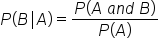The probability that event B occurs, given that event A also occurs, is the probability of both A and B occurring divided by the probability of event A.

EXAMPLE

Suppose you are rolling a standard six-sided die. What is the probability of rolling a 4?This question is not a conditional probability yet because the question is simply about the probability that the number is a 4. To find this answer, simply find the probability of rolling a 4.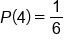Of the six possible outcomes, only one outcome would result in rolling a 4.

Suppose you are told that the number you rolled was even. What is the probability of getting a 4, given this information?
We can use the above formula that tells us that we need to divide the probability of rolling both a 4 and an even number by the probability of getting an even number. The probability of the event of getting a 4 and an even number is just 1 out of 6; there is only one die that has both characteristics. The probability of getting an even number is 3 out of 6.hint
Notice that since the fractions have the same denominator of 6, we can simplify the fraction by canceling the 6 and writing 1 over 3.

This makes sense if you consider the information we were given. If we knew that die was going to be even, this narrows our choices down to only 2, 4, or 6. Of those three outcomes, only one is a 4.term to know

Conditional Probability
The probability that one event occurs, given that another event has already occurred.

## 2. Conditional Probability Using Venn Diagrams

A Venn diagram is a useful tool to visualize conditional probability.

EXAMPLE

What is the probability of getting an even on a roulette wheel? Like the example above, this question is not a conditional probability yet because the question is simply about the probability that the number is even. To find the answer, count up all of the even sectors.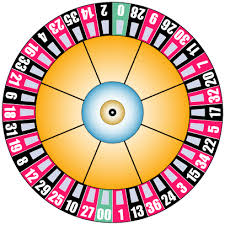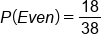hint
Notice that zero and double zero don't count as even. The evens on the roulette wheel are in two categories: even numbers that are in black and even numbers that are in red. 18 of the 38 numbers on the roulette wheel are even.

However, what if we want to know the probability that the sector is even, given that the sector is also black?
This is a conditional probability statement. We can create a Venn diagram to show the relationship between these two characteristics of even and black. Ignore any of the sectors that are neither black nor even, and the ones that are only even without being black. Some of the black numbers are also even.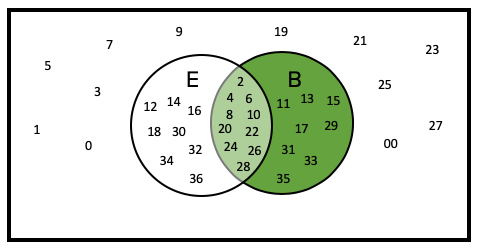Of the 18 numbers that are black on the roulette wheel, ten selections are both even and black. So the probability is 10 even black sectors out of 18 total black sectors.
We could also use the conditional probability formula which states we need to find the probability of a sector being both even and black, which is 10 out of 38, and divide by the probability of a sector being black, which is 18 out of 38.summary
Conditional probability is the probability of some second event occurring, given that some first event has already occurred. It's calculated by dividing the joint probability of the two events by the probability of the existing event (the one that's already happening). This formula works for all events. This isn't a special formula that works only for independent events or only for mutually exclusive events.

Good luck!

Source: Adapted from Sophia tutorial by Jonathan Osters.

Terms to Know
Conditional Probability

The probability that one event occurs, given that another event has already occurred.

Formulas to Know
Conditional ProbabilityRating